## Population Growth

The differential equation describing exponential growth is(1)

This can be integrated directly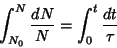(2)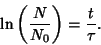(3)

Exponentiating,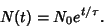(4)

Defininggives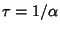in (4), so(5)

The quantityin this equation is sometimes known as the Malthusian Parameter.

Consider a more complicated growth law(6)

where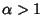is a constant. This can also be integrated directly(7)(8)(9)

Note that this expression blows up at. We are given the Initial Condition that, so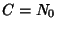.(10)

Thein the Denominator of (10) greatly suppresses the growth in the long run compared to the simple growth law.

The Logistic Growth Curve, defined by(11)

is another growth law which frequently arises in biology. It has a rather complicated solution for.

See also Gompertz Curve, Life Expectancy, Logistic Growth Curve, Lotka-Volterra Equations, Makeham Curve, Malthusian Parameter, Survivorship Curve